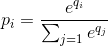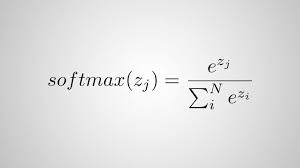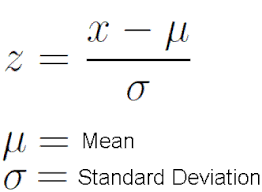2 views

In the output layer of a neural network, it is typical to use the softmax function to approximate a probability distribution:This is expensive to compute because of the exponents. Why not simply perform a Z transform so that all outputs are positive, and then normalize just by dividing all outputs by the sum of all outputs?

by (33.1k points)

Softmax as compared to standard normalization, it performs exponential normalization, that means its output directly depends upon the uniform distribution of input. While the output of normal distribution does not get affected until the ratio proportion is the same.

The formula for Softmax function:The formula for standard deviation:Example for softmax function:

>>> softmax([1,2]) # blurry image of a ferret

[0.26894142, 0.73105858]) # it is a cat perhaps !?

>>> softmax([10,20]) # crisp image of a cat

[0.0000453978687, 0.999954602]) # it is definitely a CAT !

Example for standard normalization:

>>> std_norm([1,2]) # blurry image of a ferret

[0.3333333333333333, 0.6666666666666666] # it is a cat perhaps

>>> std_norm([10,20]) # crisp image of a cat

[0.3333333333333333, 0.6666666666666666] # it is a cat perhaps !?

In the above example, softmax predicts more accurately when the image resolution is higher, but the standard normalization function predicted the same probability in lower and higher resolution of the image. That’s why Softmax is most commonly used in neural network.